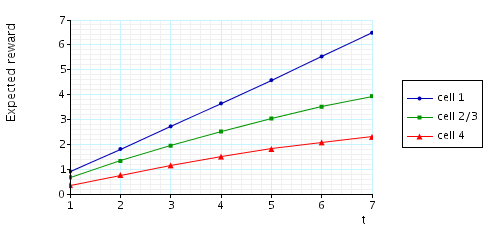# Kanban Manufacturing System

(Ciardo & Tilgner)

### Introduction:

This case study is based on the Kanban flexible manufacturing system of [CT96]. The model is represented as a CTMC. We use t to denote the number of tokens in the system. The PRISM source code for this case study given below.

```// kanban manufacturing example [CT96]
// dxp/gxn 3/2/00

ctmc

// number of tokens
const int t;

// rates
const double in1      = 1.0;
const double out4     = 0.9;
const double synch123 = 0.4;
const double synch234 = 0.5;
const double back     = 0.3;
const double redo1    = 0.36;
const double redo2    = 0.42;
const double redo3    = 0.39;
const double redo4    = 0.33;
const double ok1      = 0.84;
const double ok2      = 0.98;
const double ok3      = 0.91;
const double ok4      = 0.77;

module k1

w1 : [0..t];
x1 : [0..t];
y1 : [0..t];
z1 : [0..t];

[in]   (w1<t) & (x1<t) -> in1 	 : (w1'=w1+1) & (x1'=x1+1);
[]   (x1>0) & (y1<t) -> redo1	 : (x1'=x1-1) & (y1'=y1+1);
[]   (x1>0) & (z1<t) -> ok1 	 : (x1'=x1-1) & (z1'=z1+1);
[]   (y1>0) & (x1<t) -> back	 : (y1'=y1-1) & (x1'=x1+1);
[s1] (z1>0) & (w1>0) -> synch123 : (z1'=z1-1) & (w1'=w1-1);

endmodule

module k2

w2 : [0..t];
x2 : [0..t];
y2 : [0..t];
z2 : [0..t];

[s1] (w2<t) & (x2<t) -> 1	 : (w2'=w2+1) & (x2'=x2+1);
[]   (x2>0) & (y2<t) -> redo2 : (x2'=x2-1) & (y2'=y2+1);
[]   (x2>0) & (z2<t) -> ok2   : (x2'=x2-1) & (z2'=z2+1);
[]   (y2>0) & (x2<t) -> back  : (y2'=y2-1) & (x2'=x2+1);
[s2] (z2>0) & (w2>0) -> 1	 : (z2'=z2-1) & (w2'=w2-1);

endmodule

module k3

w3 : [0..t];
x3 : [0..t];
y3 : [0..t];
z3 : [0..t];

[s1] (w3<t) & (x3<t) -> 1	 : (w3'=w3+1) & (x3'=x3+1);
[]   (x3>0) & (y3<t) -> redo3 : (x3'=x3-1) & (y3'=y3+1);
[]   (x3>0) & (z3<t) -> ok3   : (x3'=x3-1) & (z3'=z3+1);
[]   (y3>0) & (x3<t) -> back  : (y3'=y3-1) & (x3'=x3+1);
[s2] (z3>0) & (w3>0) -> 1	 : (z3'=z3-1) & (w3'=w3-1);

endmodule

module k4

w4 : [0..t];
x4 : [0..t];
y4 : [0..t];
z4 : [0..t];

[s2] (w4<t) & (x4<t) -> synch234 : (w4'=w4+1) & (x4'=x4+1);
[]   (x4>0) & (y4<t) -> redo4	: (x4'=x4-1) & (y4'=y4+1);
[]   (x4>0) & (z4<t) -> ok4  	: (x4'=x4-1) & (z4'=z4+1);
[]   (y4>0) & (x4<t) -> back 	: (y4'=y4-1) & (x4'=x4+1);
[]   (z4>0) & (w4>0) -> out4 	: (z4'=z4-1) & (w4'=w4-1);

endmodule

// reward structures

// tokens in cell1
rewards "tokens_cell1"
true : x1+y1+z1;
endrewards

// tokens in cell2
rewards "tokens_cell2"
true : x2+y2+z2;
endrewards

// tokens in cell3
rewards "tokens_cell3"
true : x3+y3+z3;
endrewards

// tokens in cell4
rewards "tokens_cell4"
true : x4+y4+z4;
endrewards

// throughput of the system
rewards "throughput"
[in] true : 1;
endrewards

```

### Model Statistics

The table below shows statistics for each model we have built. The first section gives information about the CTMC representing the model: the number of states and the number of non zeros (transitions). The second part refers to the MTBDD which represents this CTMC: the total number of nodes, the number of leaves (terminal nodes), and the amount of memory needed to store it. The last column gives the amount of memory a sparse matrix would need to represent the same CTMC.

 t: Model: MTBDD: Sparse: States: NNZ: Nodes: Leaves: Kb: Kb: 1 160 616 499 14 9.75 7.8 2 4,600 28120 1,685 14 32.9 348 3 58,400 446,400 2,474 14 48.3 5,459 4 454,475 3,979,850 4,900 14 95.7 48,414 5 2,546,432 24,460,016 6,308 14 123 296,588 6 11,261,376 115,708,992 7,876 14 154 1,399,955 7 41,644,800 450,455,040 9,521 14 186 5,441,445

### Model Construction Times

The table below gives the times taken to construct the models. This process involves first building a CTMC (represented as an MTBDD) from the system description (in our module based language), and then computing the reachable states using a BDD based fixpoint algorithm. The total time required and the number of fixpoint iterations are given below.

 t: Construction: Time (s): Iter.s: 1 0.01 15 2 0.07 29 3 0.25 43 4 1.28 57 5 3.88 71 6 6.21 85 7 10.7 99

### Model Checking

We now report on the model checking results obtained using PRISM when verifying the following CSL properties.

```// expected number of tokens in each cell
R{"tokens_cell1"}=? [ S ]
R{"tokens_cell2"}=? [ S ]
R{"tokens_cell3"}=? [ S ]
R{"tokens_cell4"}=? [ S ]

// expected throughput of the system
R{"throughput"}=? [ S ]
```

All experiments were carried out on a 2.80GHz PC with 1 Gb memory running Linux and in all iterative methods we stop iterating when the relative error between subsequent iteration vectors is less than 1e-6, that is:The table below gives the times taken to compute the steady state probabilities using both the JOR and Gauss-Seidel methods. The number of iterations required and the time taken using the MTBDD, sparse and hybrid engines is as follows.

 N: Jacobi: Gauss Seidel: Iterations: Time per iteration: (s) Iterations: Time per iteration: (s) MTBDD Sparse Hybrid Sparse Hybrid 1 101 0.008564 0.000020 0.000020 200 0.000010 0.000010 2 166 0.574596 0.000199 0.000295 189 0.000291 0.000513 3 300 14.37466 0.003260 0.006523 206 0.004330 0.008534 4 466 - 0.029410 0.055227 323 0.037418 0.074399 5 663 - 0.179967 0.374664 461 0.222703 0.446436 6 891 - 0.898675 1.686580 622 1.075230 2.064293 7 1,148 - - 6.379109 802 - 7.844989

The graphs below present the results obtained for the different CSL properties.

• The expected number of tokens in each cell:• The expected throughput of the system: